### Image Enhancement

The above image thresholding algorithm might not always work. Sometimes you will have to do some additional processing before you can detect the location of the object and that is what we will be learning now.

In this section, we will discuss the following morphological operations that you can perform on your obtained binary image to get a more satisfactory image.

• Dilation
• Erosion
• Filling holes
• Removing small objects

Let us learn about each of them now. Please note that the definitions provided here are over simplified for easier understanding. If you want to learn more in detail, take a course on Image Processing.

We will apply all these technique on the output we got from the previous section. Note that the following image is a zoomed version as it helps to see what's happening more clearly.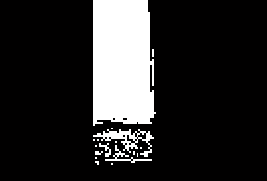#### Dilation

Dilation is the process of expanding the shapes in the image.

It can also be used when the size of the shape in binary image is smaller than the actual object. Dilation can help make the shape size bigger. Here's the syntax for dilation of an image one time.

% Dilating the image once
out2 = bwmorph(out, 'dilate');
figure, imshow(out2)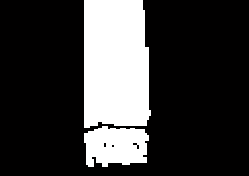You can also dilate an image multiple times by using an additional parameter as shown below.

% Dilating the image 'n' times
n = 5;
out3 = bwmorph(out, 'dilate', n);
figure, imshow(out3)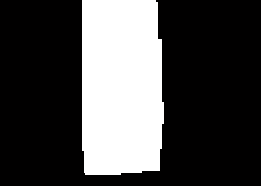The function imdilate can also be used for dilation. For learning more, type doc imdilate in your MATLAB command window.

#### Erosion

Erosion is the process of thining the object from the edges in the image. It can be used when the size of the binary image is larger than the actual object. Erosion can help make the object size smaller and filter out small object that have been unintentionally detected. Here's the syntax for erosion of an image one time.

% Eroding the image once
out2 = bwmorph(out, 'erode');
figure, imshow(out2)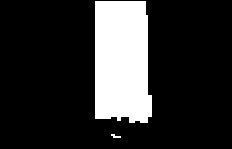You can also erode an image multiple times by using an additional parameter as shown below.

% Eroding the image 'n' times
n = 5;
out3 = bwmorph(out, 'erode', n);
figure, imshow(out3)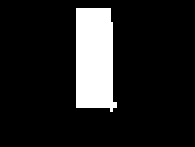#### Filling holes

Holes are black pixels in the image which are completely covered in all directions by white pixels. In other words, hole is a set of pixels that cannot be reached by filling background from edge of image. The imfill function can be used to fill the holes. An example of how it can be used is shown below.

out2 = imfill(out, 'holes'); % Filling holes
figure, imshow(out2)#### Removing small objects

Shapes in an image can be removed by using the following function. The area of the shape less than which have to be eliminated must be mentioned as one of the parameters for the function.

% remove object with area less than 100
area = 100;
out2 = bwareaopen(out, area);
figure, imshow(out2)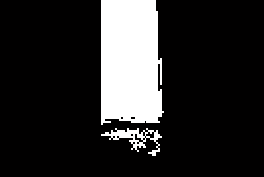Once we have enhanced the image properly, we will have only the object(s) of interest left in the image. We will learn how to find the properties of these shapes in the next section.